Courses
Courses for Kids
Free study material
Free LIVE classes
More

# Electrical Field of Charged Spherical Shell - JEE## What are Gauss Law and Gaussian Surface?

In order to understand the electrical behaviour of a charged spherical shell, Gauss Law is important. It is applied to not just electrical fields due to a charged spherical shell, but also to charged plates and cylinders etc. According to Gauss Law, the net electric flux passing through any hypothetical closed surface is equal to $\left( \frac{1}{{{\varepsilon }_{\circ }}} \right)$ time the net electric charge present within that surface. The hypothetical closed surface is called the Gaussian surface. In this section, we will discuss finding the electric field and electric potential at various points in a charged sphere.

## Self-Energy of Charged Conductors

Spherical shells are usually charged by assembling charges from an infinite distance on points of the spherical shell. In order to assemble these charges, work is to be done. And this work is usually stored in the form of self-energy on the charged spherical shells. For instance, no opposition will be faced in bringing the first charge from an infinite distance. However, when a second charge is brought from a distance, an opposition will be offered by the existing charge and hence more energy is required. Similarly, the energy required to bring a charge will be directly proportional to the number of charges already present.

Hence, the self-energy of a charged shell varies indirectly with respect to the distance from which the charges have to be brought to the centre of the shell, i.e., if the charges are to be brought from a far distance, then more work is to be done, and less self-energy is stored. Likewise for charges to be brought from nearby, comparatively less work is enough and hence higher is the self-energy.

The equation for self-energy can be derived as follows:

$U = \frac{Q^{2}}{8\pi \varepsilon _{o}R}$

## Electric Field and Potential due to a Charged  Spherical Shell

For a charged spherical shell with a charge q and radius R, let us find the electric field and potential inside, at the centre, and outside the sphere can be found using Gauss Law. Let us derive the electric field and potential due to the charged spherical shell.

At a Point Outside the Charged Spherical Shell (r>R)

Let the point be at a distance r from the centre of the sphere.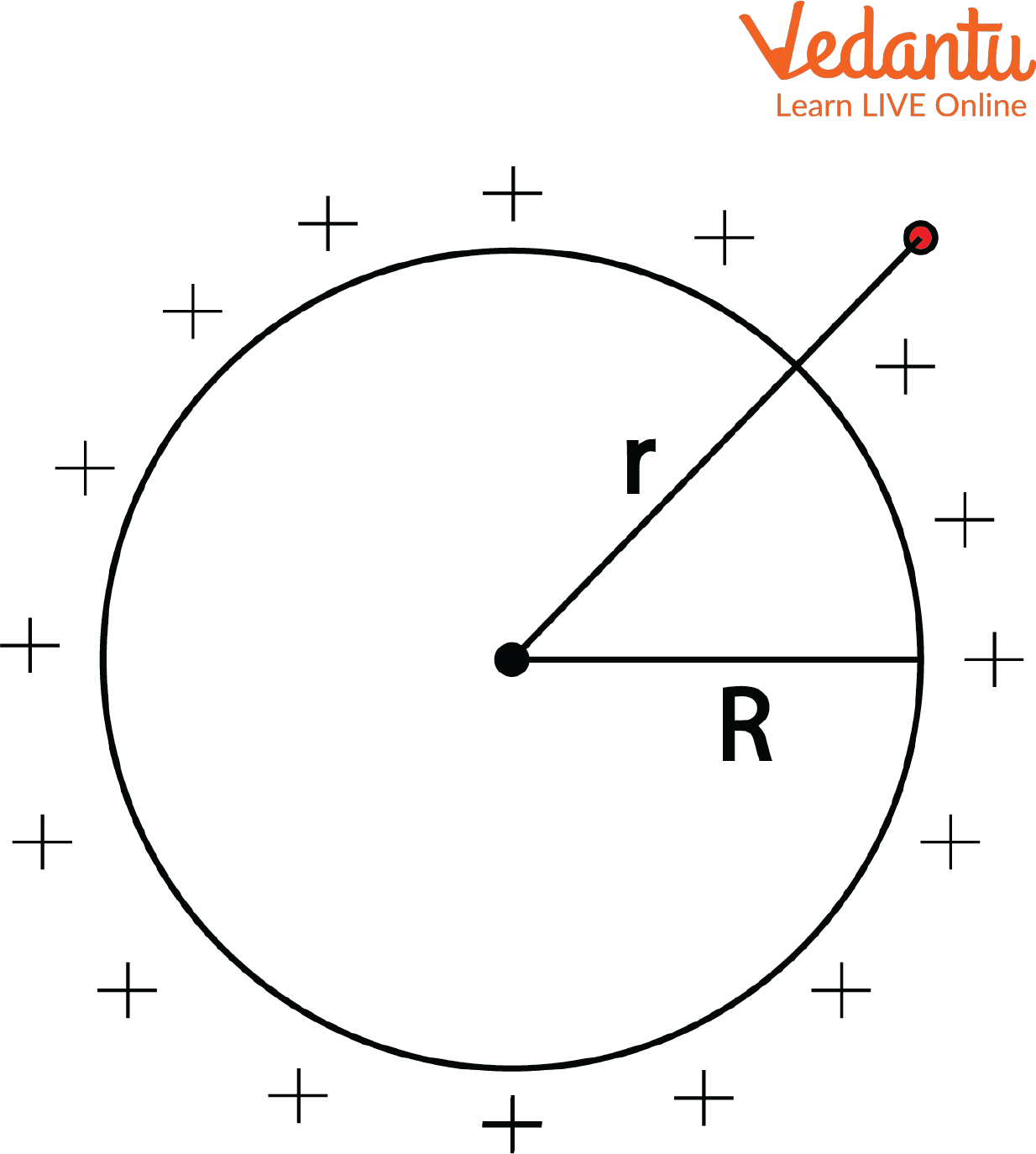At a Point Outside the Charged Spherical Shell (r>R)

The electric field intensity at a point on the surface of the charged non-conducting sphere is:

$\overrightarrow{E} = \frac{1}{4\pi \epsilon _{o}}\frac{q}{r^{2}}\hat{r} (r>R)$

The formula for finding the potential at this point is given by

$V=-\int_{\infty }^{r}{{\vec{E}}}.\overrightarrow{dr}$

$V=-\int_{\infty }^{r}{\frac{1}{4\pi {{\epsilon }_{o}}}}\frac{q}{{{r}^{2}}}\hat{r}.\widehat{dr}$

$V=-\frac{q}{4\pi {{\epsilon }_{o}}}\int_{\infty }^{r}{\frac{1}{{{r}^{2}}}}dr$

$V=-\frac{q}{4\pi {{\epsilon }_{o}}}\left[ \frac{-1}{r} \right]_{\infty }^{r}$

$V=\frac{q}{4\pi {{\epsilon }_{o}}}\left[ \frac{1}{r}-\frac{1}{\infty } \right]_{\infty }^{r}$

Since $\frac{1}{\infty }=0$ ,

$V = \frac{q}{4\pi \epsilon _{o}r}$                                               .....(1)

Hence, the electric potential for points outside the non-conducting sphere is inversely proportional to their distance from the centre of the sphere.

At a point on the surface of the charged spherical shell (r=R)

Let the point be on the surface of the charged sphere, i.e., at a distance R from the centre.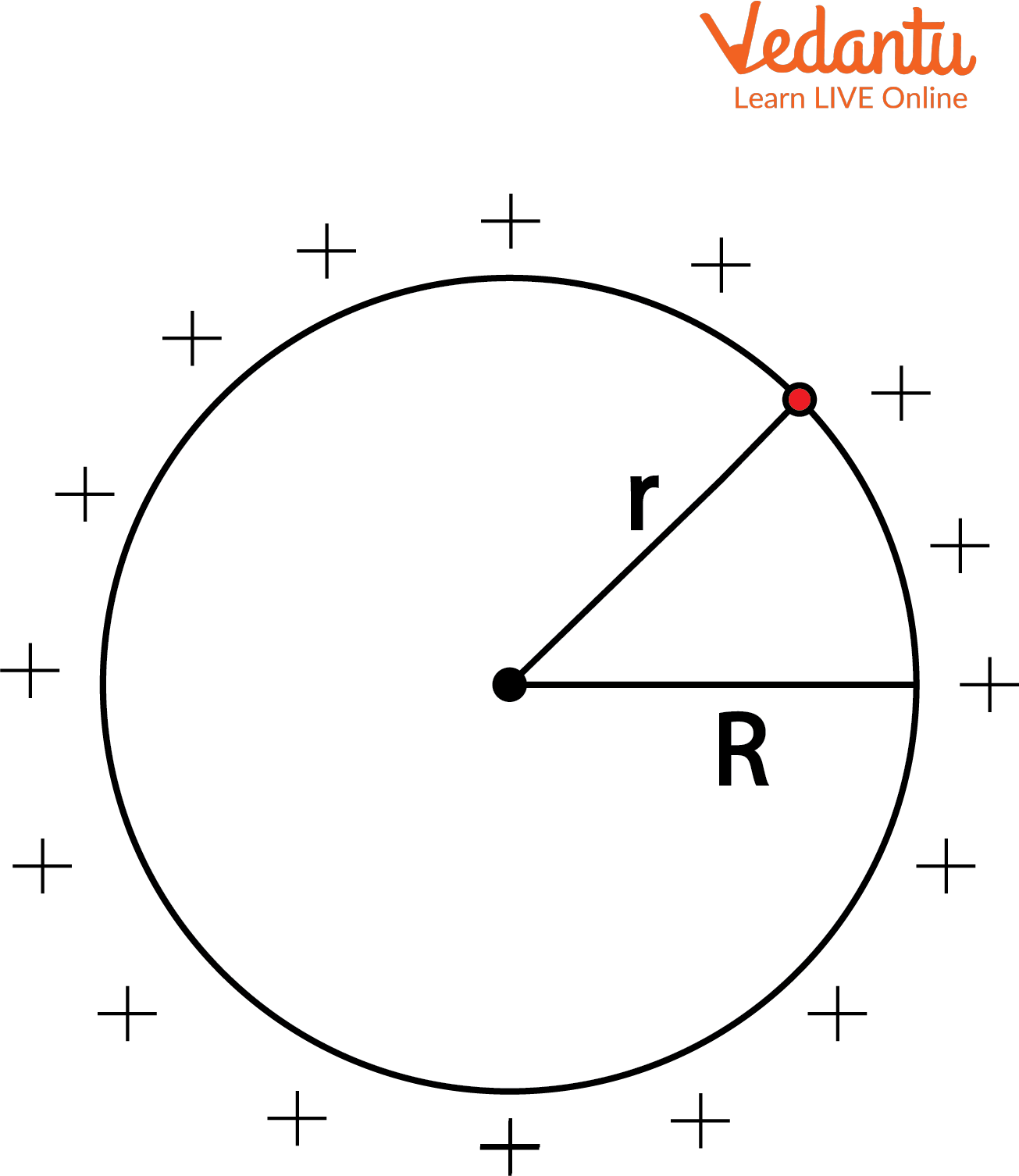Point on the Surface of a Charged Spherical Shell

The electric field intensity at a point on the surface of the charged non-conducting sphere is given by replacing r = R in the equation (1)

$\vec{E}=\frac{1}{4\pi {{\epsilon }_{o}}}\frac{q}{{{R}^{2}}}\hat{r}$   (r=R) and

$V = \frac{q}{4\pi \epsilon _{o}R}$                                 .....(2)

At a Point Inside the Charged Spherical Shell (r<R)

Let the point be inside the charged sphere at a distance (r < R) from the centre. And we know that at any point inside the shell, the electric field E = 0. Thus, the total work done in bringing a unit positive charge from a point on the surface to any point inside the charged shell will be zero. Thus, the electric potential has a definite value at all points within the spherical shell and is equal to the potential at the surface.

This can be proved with the help of the relation between the electric potential and the electric field, i.e.,

$\vec E= - \dfrac {dV}{dr}$

$\dfrac {dV}{dr}=0$

$\therefore, V= Constant$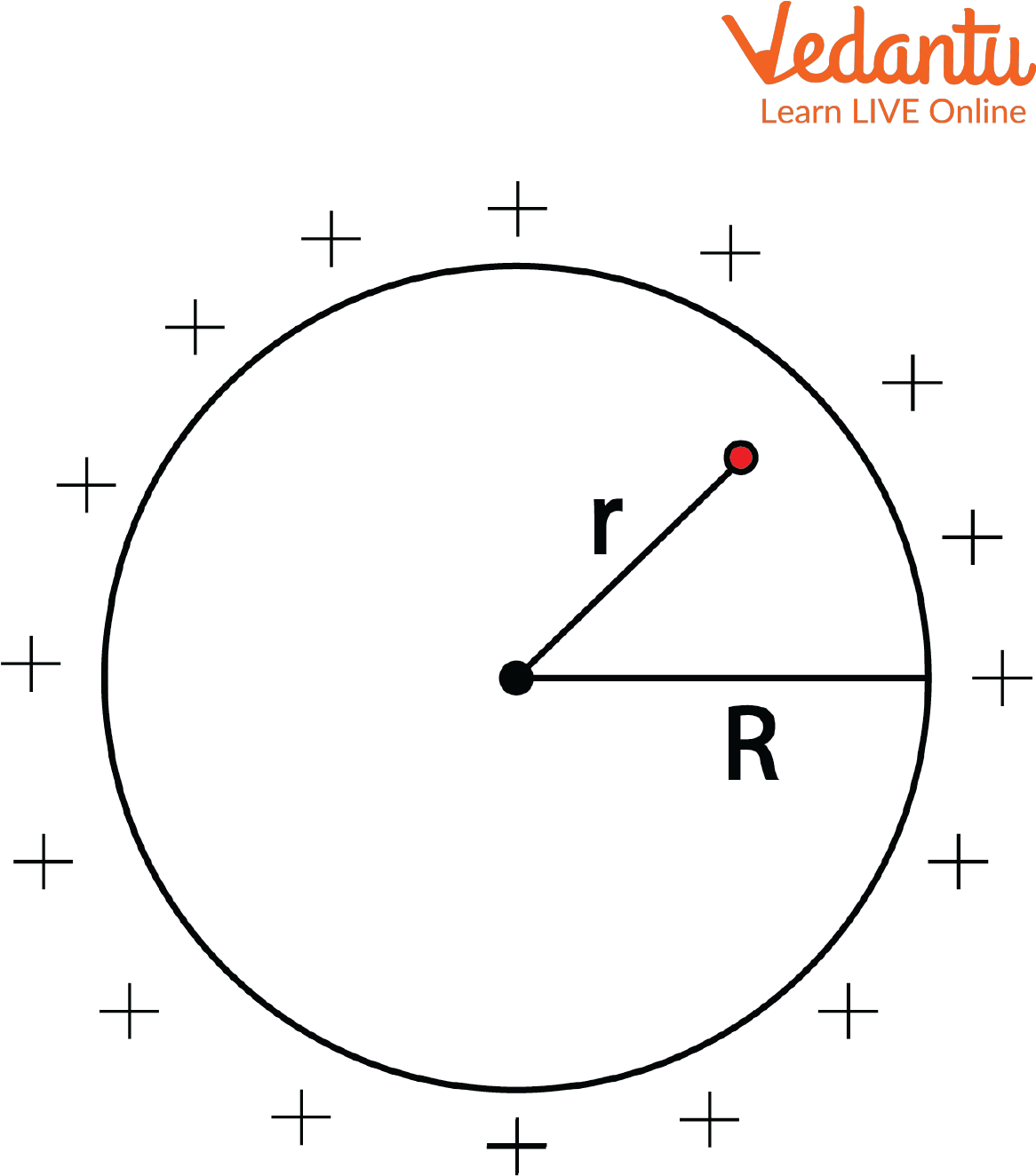Point inside the Charged Spherical Shell

Now let us derive the electric field and potential:

$\vec {E}=\frac{1}{4\pi \epsilon _{o}} \frac{q}{r^{2}} (r < R)$

Thus,

$V=\left[ -\int\limits_{\infty }^{R}{\overrightarrow{E.}\overrightarrow{dr}} \right]+\left[ -\int\limits_{R }^{r}{\overrightarrow{E.}\overrightarrow{dr}} \right]$

$V=\frac{q}{4\pi {{\varepsilon }_{\circ }}R}-0$

(Inside the charged shell, the value of the electric field will be zero, i.e., E=0)

$V=\frac{q}{4\pi {{\varepsilon }_{\circ }}R}$

Therefore, the electric potential has a constant value at all points within the spherical shell and is having the same value as the potential at the surface of the charged spherical shell.

So, the graphical representation of the variation of potential with respect to distance r can be represented as shown below.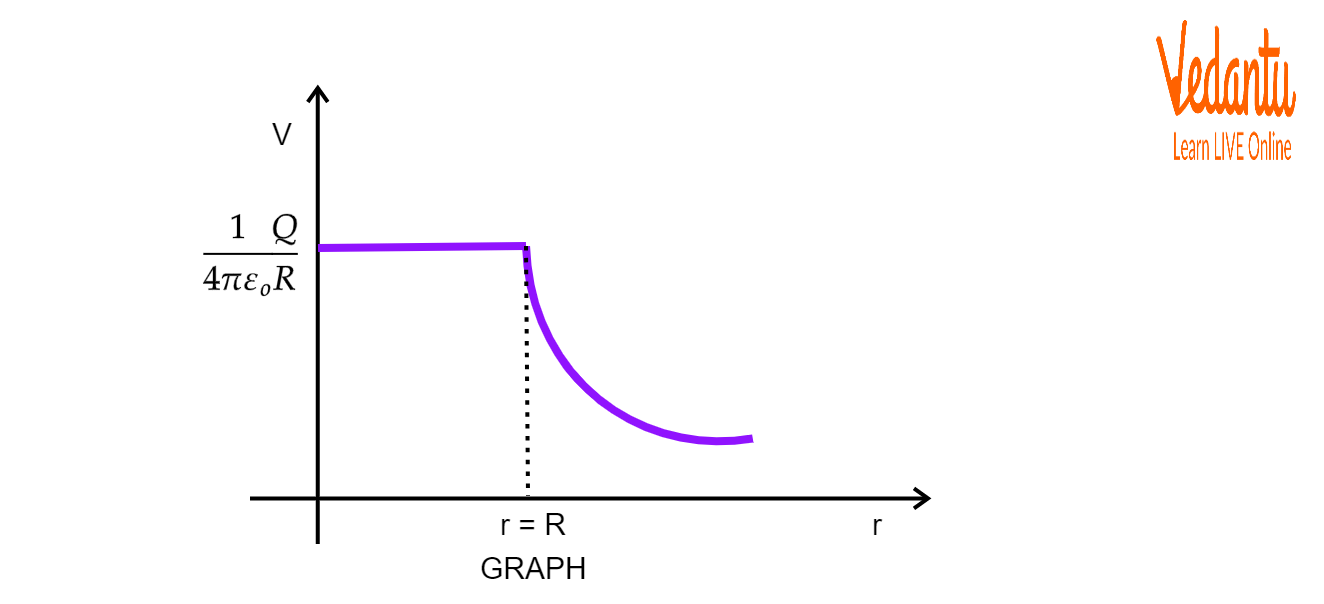Graphical Representation of Variation of Potential with Distance ‘r’

Variation in Electric Potential at Various Points for a Non-Conducting Charged Sphere

Without going into detailed derivation, let us discuss the cases of a non-conducting charged sphere.

For a point inside the sphere, the electric field intensity is given as:

$E=\frac{r\rho }{3\varepsilon }$

Here, $\varepsilon$ is the permittivity of the material of the sphere, r denotes the distance of the point from the centre and less than R, the sphere radius, and $\rho$ is the charge density. The relation gives a clear picture that the electric field intensity inside is directly proportional to the distance r from the centre.

At r = 0, that means at the centre of the sphere, the electric field intensity is zero.

At r = R, that is on the surface of the non-conducting charged sphere, the electric field intensity is

$E=\frac{\rho R}{3\varepsilon }=\text{maximum}$

At a point outside the sphere, i.e., r > R, the electric field density is

$E=\frac{q}{4\pi {{\varepsilon }_{0}}{{r}^{2}}}$

Here, we can see that the electric field intensity drops as the distance r increases following an inverse square law. In a non-conducting charged sphere, the charges are present on the inside surface of the sphere as well. So, the electric potential is also present inside the sphere. The following diagram depicts the change in electric potential at various points for a charged non-conducting sphere.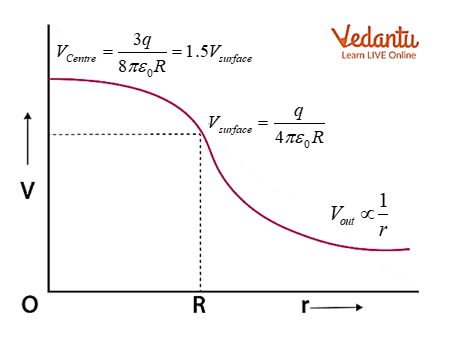Variation in Electric Potential

## Summary

A charged sphere that is not a conductor by nature has maximum electric potential at the centre of the sphere which is 1.5 times the electric potential at the surface of the non-conducting sphere. Outside the surface of the sphere, the electric potential varies indirectly with respect to the distance from the centre. Similarly, for a conducting sphere that is charged, the electric field is zero at points inside the sphere and the potential is constant. At the surface of the charged conducting sphere, the electric field is maximum and henceforth decreases as the distance increases from the surface. Also, the electric potential at points on and outside the surface is the same as that of a point charge.

Last updated date: 25th May 2023
Total views: 88.8k
Views today: 0.47k

## FAQs on Electrical Field of Charged Spherical Shell - JEE

1. Why is the electrostatic potential constant throughout the conductors’ volume and has the value the same as that at the surface?

The major reasons are as follows:

• The electrostatic field inside a conductor is zero.

• The electrostatic field is normal to the surface at points on the surface of a charged conductor.

Due to these two reasons, no or very negligible amount of work is done in moving a test charge on the surface and within the conductor. In simpler terms, the potential difference between two points on the surface and within the conductor is zero. Hence, the electrostatic potential is constant throughout the volume of the conductor and the value is the same as that at the surface.

2. State few applications of a charged sphere.

A very large amount of charge can be easily deposited on the sphere because the movement of charges to the surface of the sphere is very quick. In spite of the limitation due to ionisation of surrounding elements, and the generation of free charges that neutralise the extra charges, charged spheres are good for collecting and storing charges.